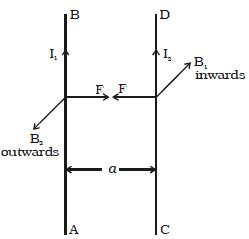# Experiment: Force between two long Parallel Current Carrying Conductors

The force between two long parallel current-carrying conductors

AB and CD are two straight very long parallel conductors placed in the air at a distance a. They carry currents I1 and I2 respectively. (Figure). The magnetic induction due to current I1 in AB at a distance a is

B1 = (μ0I1/2πα) … … … (1)Fig: Force between two long parallel current-carrying conductors

Consider the system shown in the figure above. Here, we have two parallel Current carrying conductors, separated by a distance ‘d’, such that one of the conductors is carrying a current I1 and the other is carrying I2, as shown in the figure. This magnetic field acts perpendicular to the plane of the paper and inwards. The conductor CD with current I2 is situated in this magnetic field. Hence, the force on a segment of length l of CD due to magnetic field B1 is

F = B1I2l

substituting equation (1)

F = (μ0I1 I2l / 2πα) … … … (2)

By Fleming’s Left-Hand Rule, F acts towards left. Similarly, the magnetic induction due to current I2 flowing in CD at a distance a is

B2 = (μ0I2/2πα) … … … (3)

This magnetic field acts perpendicular to the plane of the paper and outwards. The conductor AB with current I1, is situated in this field. Hence force on a segment of length l of AB due to magnetic field B2 is

F = B2I2l

substituting equation (3)

F = (μ0I1 I2l / 2πα) … … … (4)

By Fleming’s left-hand rule, this force acts towards the right. These two forces are given in equations (2) and (4) attract each other. Hence, two parallel wires carrying currents in the same direction attract each other and if they carry currents in the opposite direction, repel each other.

From the knowledge gained before, we can say that the conductor 2 experiences the same magnetic field at every point along its length due to the conductor 1. The direction of the magnetic force is indicated in the figure and is found using the right-hand thumb rule. The direction of the magnetic field, as we can see, is downwards due to the first conductor.

We know the existence of a magnetic field due to a current-carrying conductor and the Biot – Savart’s law. And an external magnetic field exerts a force on a current-carrying conductor and the Lorentz force formula that governs this principle. Thus, from the two studies, we can say that any two current-carrying conductors when placed near each other, will exert a magnetic force on each other.